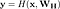# Highway Networks with TensorFlow

This week I implemented highway networks to get an intuition for how they work. Highway networks, inspired by LSTMs, are a method of constructing networks with hundreds, even thousands, of layers. Let’s see how we construct them using TensorFlow.

## Implementation

For comparison, let’s start with a standard fully-connected (or “dense”) layer. We need a weight matrix and a bias vector then we’ll compute the following for the layer output:Computing the output of a dense layer. (Bias omitted for simplicity and to match the paper.)

Here’s what a dense layer looks like as a graph in TensorBoard:

For the highway layer what we want are two “gates” that control the flow of information. The “transform” gate controls how much of the activation we pass through and the “carry” gate controls how much of the unmodified input we pass through. Otherwise, the layer largely resembles a dense layer with a few additions:Computing the highway layer output. (Bias omitted for simplicity and to match the paper.)
• An extra set of weights and biases to be learned for the gates.
• The transform gate operation (T).
• The carry gate operation (C or just 1 - T).
• The layer output (y) with the new gates.

What happens is that when the transform gate is 1, we pass through our activation (H) and suppress the carry gate (since it will be 0). When the carry gate is 1, we pass through the unmodified input (x), while the activation is suppressed.

Here’s what the highway layer graph looks in TensorBoard:

Using a highway layer in a network is also straightforward. One detail to keep in mind is that consecutive highway layers must be the same size but you can use fully-connected layers to change dimensionality. This becomes especially complicated in convolutional layers where each layer can change the output dimensions. We can use padding (‘SAME’) to maintain each layers dimensionality.

Otherwise, by simply using hyperparameters from the TensorFlow docs (i.e. no hyperparameter search) the fully-connected highway network performed much better than a fully-connected network. Using MNIST as my simple trial:

• 20 fully-connected layers fail to achieve more than 15% accuracy.
• 18 highway layers (with two fully-connected layers to transform the input and output) achieves ~95% accuracy. Which is also much better than a shallow network which only reaches 91%.

Now that we have a highway network, I wanted to answer a few questions that came up for me while reading the paper. For instance, how deep will the network converge? The paper briefly mentions 1000 layers:

In pilot experiments, SGD did not stall for networks with more than 1000 layers. (2.2)

Can we train with 1000 layers on MNIST?

Yes, also reaching around 95% accuracy. Try it out with a carry bias around -20.0 for MNIST (from the paper the network will only utilize ~15 layers anyway). The network can probably even go deeper since the it’s just learning to carry the last 980 layers or so. We can’t do much useful at or past 1000 layers so that seems sufficient for now.

What happens if you set very low or very high carry biases?

In either extreme the network simply fails to converge in a reasonable amount of time. In the case of low biases (more positive), the network starts as if the carry gates aren’t present at all. In the case of high biases (more negative), we’re putting more emphasis on carrying and the network can take a long time to overcome that. Otherwise, the biases don’t seem to need to be exact, at least on this simple example. When in doubt start with high biases (more negative) since it’s easier to learn to overcome carrying than without carry gates (which is just a plain network).

## Conclusion

Overall I was happy with how easy highway networks were to implement. They’re fully differentiable with only a single additional hyperparameter for the initial carry bias. One downside is that highway layers do require additional parameters for the transform weights and biases. However, since we can go deeper, the layers do not need to be as wide which can compensate.

Here’s are the complete notebooks if you want to play with the code: fully-connected highway repo and convolutional highway repo.